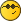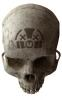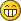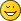How to calculate sun position?buuuudzikSenior Member Posts: 871 Threads: 150 Joined: Jul 2015 Reputation: 27 13.12.2015, 16:23 Hello Do you have some algorithm to calculate a sun position?adminAdministrator Posts: 3363 Threads: 21 Joined: Jun 2015 Reputation: 174 14.12.2015, 08:07 This function should work, though it was not tested much: Code:```-- solar altitude, azimuth (degrees) function sunposition(latitude, longitude, time)   time = time or os.time()   if type(time) == 'table' then     time = os.time(time)   end   local date = os.date('*t', time)   local timezone = (os.time(date) - os.time(os.date('!*t', time))) / 3600   if date.isdst then     timezone = timezone + 1   end   local utcdate = os.date('*t', time - timezone * 3600)   local latrad = math.rad(latitude)   local fd = (utcdate.hour + utcdate.min / 60 + utcdate.sec / 3600) / 24   local g = (2 * math.pi / 365.25) * (utcdate.yday + fd)   local d = math.rad(0.396372 - 22.91327 * math.cos(g) + 4.02543 * math.sin(g) - 0.387205 * math.cos(2 * g)           + 0.051967 * math.sin(2 * g) - 0.154527 * math.cos(3 * g) + 0.084798 * math.sin(3 * g))   local t = math.rad(0.004297 + 0.107029 * math.cos(g) - 1.837877 * math.sin(g)           - 0.837378 * math.cos(2 * g) - 2.340475 * math.sin(2 * g))   local sha = 2 * math.pi * (fd - 0.5) + t + math.rad(longitude)   local sza = math.acos(math.sin(latrad) * math.sin(d) + math.cos(latrad) * math.cos(d) * math.cos(sha))   local saa = math.acos((math.sin(d) - math.sin(latrad) * math.cos(sza)) / (math.cos(latrad) * math.sin(sza)))   return 90 - math.deg(sza), math.deg(saa) end```buuuudzikSenior Member Posts: 871 Threads: 150 Joined: Jul 2015 Reputation: 27 14.12.2015, 09:50 (14.12.2015, 08:07)admin Wrote: This function should work, though it was not tested much: Code:```-- solar altitude, azimuth (degrees) function sunposition(latitude, longitude, time)  time = time or os.time()  if type(time) == 'table' then    time = os.time(time)  end  local date = os.date('*t', time)  local timezone = (os.time(date) - os.time(os.date('!*t', time))) / 3600  if date.isdst then    timezone = timezone + 1  end  local utcdate = os.date('*t', time - timezone * 3600)  local latrad = math.rad(latitude)  local fd = (utcdate.hour + utcdate.min / 60 + utcdate.sec / 3600) / 24  local g = (2 * math.pi / 365.25) * (utcdate.yday + fd)  local d = math.rad(0.396372 - 22.91327 * math.cos(g) + 4.02543 * math.sin(g) - 0.387205 * math.cos(2 * g)          + 0.051967 * math.sin(2 * g) - 0.154527 * math.cos(3 * g) + 0.084798 * math.sin(3 * g))  local t = math.rad(0.004297 + 0.107029 * math.cos(g) - 1.837877 * math.sin(g)          - 0.837378 * math.cos(2 * g) - 2.340475 * math.sin(2 * g))  local sha = 2 * math.pi * (fd - 0.5) + t + math.rad(longitude)  local sza = math.acos(math.sin(latrad) * math.sin(d) + math.cos(latrad) * math.cos(d) * math.cos(sha))  local saa = math.acos((math.sin(d) - math.sin(latrad) * math.cos(sza)) / (math.cos(latrad) * math.sin(sza)))  return 90 - math.deg(sza), math.deg(saa) end``` It works. Thank you I create a library with this function in User library and in resident script I add this script: Code:```latitude = 50.990 longtitude = -23.179 time = nil altitude, azimuth = sunposition(latitude, longitude, time) log(altitude, azimuth)``` Result is very close to the results from online calculators.adminAdministrator Posts: 3363 Threads: 21 Joined: Jun 2015 Reputation: 174 14.12.2015, 10:20 Yes, I think it was ported from JavaScript based on one of online calculators.oab_norJunior Member Posts: 6 Threads: 1 Joined: Aug 2015 Reputation: 1 14.12.2015, 14:18 Hi, while we’re on this topic.  Has anyone tried to work out an algorithm to calculate slate position of venetian blinds? Given the orientation of a window/blind, and the solar altitude and azimuth (given in post above).  What is the optimal slate position to avoid direct sunlight?I’we been playing with some calculations. Even tried to “reverse engineer” observations from a major brand “KNX Wetterstation”, but never really got hold of it. Anyone with good experiences on this? Any suggestions on how to approach this is welcome.  E.g. Is there one formula?  Should i use a lookup table with different altitude- intervals for different azimuth “ranges”?  Should i use a combination?adminAdministrator Posts: 3363 Threads: 21 Joined: Jun 2015 Reputation: 174 15.12.2015, 13:24 For starters, you can try with just using altitude since blinds only move on one axis. Blinds should be perpendicular to current sun position. Then you can tweak it by checking azimuth so you don't adjust blinds when there's no direct sunlight.oab_norJunior Member Posts: 6 Threads: 1 Joined: Aug 2015 Reputation: 1 16.12.2015, 08:47 (15.12.2015, 13:24)admin Wrote: For starters, you can try with just using altitude since blinds only move on one axis. Blinds should be perpendicular to current sun position. Then you can tweak it by checking azimuth so you don't adjust blinds when there's no direct sunlight. Tanks, maybe  i'm just making it too complex.   There should, of course, be a 1:1 relation between sun altitude and  slate position (e.g. 0-100%) – regardless of azimuth and orientation of the blinds/window.buuuudzikSenior Member Posts: 871 Threads: 150 Joined: Jul 2015 Reputation: 27 20.12.2015, 15:03 Can I add this library to LM? https://github.com/mourner/suncalcadminAdministrator Posts: 3363 Threads: 21 Joined: Jun 2015 Reputation: 174 21.12.2015, 07:08 You cannot use this library directly, but rewriting it in Lua should be quite easy.Trond HoyemMember Posts: 116 Threads: 19 Joined: Feb 2017 Reputation: 3 09.05.2017, 12:22 (14.12.2015, 08:07)admin Wrote: This function should work, though it was not tested much: Code:```-- solar altitude, azimuth (degrees) function sunposition(latitude, longitude, time)  time = time or os.time()  if type(time) == 'table' then    time = os.time(time)  end  local date = os.date('*t', time)  local timezone = (os.time(date) - os.time(os.date('!*t', time))) / 3600  if date.isdst then    timezone = timezone + 1  end  local utcdate = os.date('*t', time - timezone * 3600)  local latrad = math.rad(latitude)  local fd = (utcdate.hour + utcdate.min / 60 + utcdate.sec / 3600) / 24  local g = (2 * math.pi / 365.25) * (utcdate.yday + fd)  local d = math.rad(0.396372 - 22.91327 * math.cos(g) + 4.02543 * math.sin(g) - 0.387205 * math.cos(2 * g)          + 0.051967 * math.sin(2 * g) - 0.154527 * math.cos(3 * g) + 0.084798 * math.sin(3 * g))  local t = math.rad(0.004297 + 0.107029 * math.cos(g) - 1.837877 * math.sin(g)          - 0.837378 * math.cos(2 * g) - 2.340475 * math.sin(2 * g))  local sha = 2 * math.pi * (fd - 0.5) + t + math.rad(longitude)  local sza = math.acos(math.sin(latrad) * math.sin(d) + math.cos(latrad) * math.cos(d) * math.cos(sha))  local saa = math.acos((math.sin(d) - math.sin(latrad) * math.cos(sza)) / (math.cos(latrad) * math.sin(sza)))  return 90 - math.deg(sza), math.deg(saa) end```Hi I used this today. What I see is that when the azimuth get to 180, it starts counting down rather than going on to the full 360... Any ideas why? There are 10 kinds of people in the world; those who can read binary and those who don'tadminAdministrator Posts: 3363 Threads: 21 Joined: Jun 2015 Reputation: 174 11.05.2017, 07:49 Try this one instead: Code:```function suncalc(lat, lng, time)   -- sun calculations are based on http://aa.quae.nl/en/reken/zonpositie.html formulas   local PI   = math.pi   local sin  = math.sin   local cos  = math.cos   local tan  = math.tan   local asin = math.asin   local atan = math.atan2   local acos = math.acos   local deg  = math.deg   local rad  = PI / 180   local e = rad * 23.4397 -- obliquity of the Earth   local daysec = 60 * 60 * 24   local J1970 = 2440588   local J2000 = 2451545   local function toDays(time)     return time / daysec - 0.5 + J1970 - J2000   end   local function rightAscension(l, b)     return atan(sin(l) * cos(e) - tan(b) * sin(e), cos(l))   end   local function declination(l, b)     return asin(sin(b) * cos(e) + cos(b) * sin(e) * sin(l))   end   local function azimuth(H, phi, dec)     return atan(sin(H), cos(H) * sin(phi) - tan(dec) * cos(phi))   end   local function altitude(H, phi, dec)     return asin(sin(phi) * sin(dec) + cos(phi) * cos(dec) * cos(H))   end   local function siderealTime(d, lw)     return rad * (280.16 + 360.9856235 * d) - lw   end   local function astroRefraction(h)     if h < 0 then -- the following formula works for positive altitudes only.       h = 0 -- if h = -0.08901179 a div/0 would occur.     end     -- formula 16.4 of "Astronomical Algorithms" 2nd edition by Jean Meeus (Willmann-Bell, Richmond) 1998.     -- 1.02 / tan(h + 10.26 / (h + 5.10)) h in degrees, result in arc minutes -> converted to rad:     return 0.0002967 / math.tan(h + 0.00312536 / (h + 0.08901179))   end   -- general sun calculations   local function solarMeanAnomaly(d)     return rad * (357.5291 + 0.98560028 * d)   end   local function eclipticLongitude(M)     local C = rad * (1.9148 * sin(M) + 0.02 * sin(2 * M) + 0.0003 * sin(3 * M)) -- equation of center     local P = rad * 102.9372 -- perihelion of the Earth     return M + C + P + PI   end   local function sunCoords(d)     local M = solarMeanAnomaly(d)     local L = eclipticLongitude(M)     return declination(L, 0), rightAscension(L, 0)   end   local lw      = rad * -lng   local phi     = rad * lat   local d       = toDays(time or os.time())   local dec, ra = sunCoords(d)   local H       = siderealTime(d, lw) - ra   local alt, az = altitude(H, phi, dec), azimuth(H, phi, dec)   return deg(alt), 180 + deg(az) end```Trond HoyemMember Posts: 116 Threads: 19 Joined: Feb 2017 Reputation: 3 21.05.2017, 20:59 (11.05.2017, 07:49)admin Wrote: Try this one instead: Code:```function suncalc(lat, lng, time)  -- sun calculations are based on http://aa.quae.nl/en/reken/zonpositie.html formulas  local PI   = math.pi  local sin  = math.sin  local cos  = math.cos  local tan  = math.tan  local asin = math.asin  local atan = math.atan2  local acos = math.acos  local deg  = math.deg  local rad  = PI / 180  local e = rad * 23.4397 -- obliquity of the Earth  local daysec = 60 * 60 * 24  local J1970 = 2440588  local J2000 = 2451545  local function toDays(time)    return time / daysec - 0.5 + J1970 - J2000  end  local function rightAscension(l, b)    return atan(sin(l) * cos(e) - tan(b) * sin(e), cos(l))  end  local function declination(l, b)    return asin(sin(b) * cos(e) + cos(b) * sin(e) * sin(l))  end  local function azimuth(H, phi, dec)    return atan(sin(H), cos(H) * sin(phi) - tan(dec) * cos(phi))  end  local function altitude(H, phi, dec)    return asin(sin(phi) * sin(dec) + cos(phi) * cos(dec) * cos(H))  end  local function siderealTime(d, lw)    return rad * (280.16 + 360.9856235 * d) - lw  end  local function astroRefraction(h)    if h < 0 then -- the following formula works for positive altitudes only.      h = 0 -- if h = -0.08901179 a div/0 would occur.    end    -- formula 16.4 of "Astronomical Algorithms" 2nd edition by Jean Meeus (Willmann-Bell, Richmond) 1998.    -- 1.02 / tan(h + 10.26 / (h + 5.10)) h in degrees, result in arc minutes -> converted to rad:    return 0.0002967 / math.tan(h + 0.00312536 / (h + 0.08901179))  end  -- general sun calculations  local function solarMeanAnomaly(d)    return rad * (357.5291 + 0.98560028 * d)  end  local function eclipticLongitude(M)    local C = rad * (1.9148 * sin(M) + 0.02 * sin(2 * M) + 0.0003 * sin(3 * M)) -- equation of center    local P = rad * 102.9372 -- perihelion of the Earth    return M + C + P + PI  end  local function sunCoords(d)    local M = solarMeanAnomaly(d)    local L = eclipticLongitude(M)    return declination(L, 0), rightAscension(L, 0)  end  local lw      = rad * -lng  local phi     = rad * lat  local d       = toDays(time or os.time())  local dec, ra = sunCoords(d)  local H       = siderealTime(d, lw) - ra  local alt, az = altitude(H, phi, dec), azimuth(H, phi, dec)  return deg(alt), 180 + deg(az) end``` Thanks. That one worked. Trond There are 10 kinds of people in the world; those who can read binary and those who don'tAuzziiJunior Member Posts: 20 Threads: 9 Joined: Sep 2015 Reputation: 0 30.10.2017, 13:58 (21.05.2017, 20:59)Trond Hoyem Wrote: (11.05.2017, 07:49)admin Wrote: Try this one instead: Code:```function suncalc(lat, lng, time)  -- sun calculations are based on http://aa.quae.nl/en/reken/zonpositie.html formulas  local PI   = math.pi  local sin  = math.sin  local cos  = math.cos  local tan  = math.tan  local asin = math.asin  local atan = math.atan2  local acos = math.acos  local deg  = math.deg  local rad  = PI / 180  local e = rad * 23.4397 -- obliquity of the Earth  local daysec = 60 * 60 * 24  local J1970 = 2440588  local J2000 = 2451545  local function toDays(time)    return time / daysec - 0.5 + J1970 - J2000  end  local function rightAscension(l, b)    return atan(sin(l) * cos(e) - tan(b) * sin(e), cos(l))  end  local function declination(l, b)    return asin(sin(b) * cos(e) + cos(b) * sin(e) * sin(l))  end  local function azimuth(H, phi, dec)    return atan(sin(H), cos(H) * sin(phi) - tan(dec) * cos(phi))  end  local function altitude(H, phi, dec)    return asin(sin(phi) * sin(dec) + cos(phi) * cos(dec) * cos(H))  end  local function siderealTime(d, lw)    return rad * (280.16 + 360.9856235 * d) - lw  end  local function astroRefraction(h)    if h < 0 then -- the following formula works for positive altitudes only.      h = 0 -- if h = -0.08901179 a div/0 would occur.    end    -- formula 16.4 of "Astronomical Algorithms" 2nd edition by Jean Meeus (Willmann-Bell, Richmond) 1998.    -- 1.02 / tan(h + 10.26 / (h + 5.10)) h in degrees, result in arc minutes -> converted to rad:    return 0.0002967 / math.tan(h + 0.00312536 / (h + 0.08901179))  end  -- general sun calculations  local function solarMeanAnomaly(d)    return rad * (357.5291 + 0.98560028 * d)  end  local function eclipticLongitude(M)    local C = rad * (1.9148 * sin(M) + 0.02 * sin(2 * M) + 0.0003 * sin(3 * M)) -- equation of center    local P = rad * 102.9372 -- perihelion of the Earth    return M + C + P + PI  end  local function sunCoords(d)    local M = solarMeanAnomaly(d)    local L = eclipticLongitude(M)    return declination(L, 0), rightAscension(L, 0)  end  local lw      = rad * -lng  local phi     = rad * lat  local d       = toDays(time or os.time())  local dec, ra = sunCoords(d)  local H       = siderealTime(d, lw) - ra  local alt, az = altitude(H, phi, dec), azimuth(H, phi, dec)  return deg(alt), 180 + deg(az) end``` Thanks. That one worked. Trond Hi, Maybe someone have example code? I need to get sun position every 5 min. 1) Elevation degree (0-100%) 2) Azimuth (0-360*) thx Attached Files Image(s)Erwin van der ZwartSenior Member Posts: 1443 Threads: 4 Joined: Jul 2015 Reputation: 80 30.10.2017, 15:19 (This post was last modified: 30.10.2017, 15:20 by Erwin van der Zwart.) Hi, Use function suncalc() like this: Code:```lat = 52.5167747 -- Zwolle - Netherlands lng = 6.0830219 -- Zwolle - Netherlands time = os.time() alt, az = suncalc(lat, lng, time) grp.update('33/1/1', alt) grp.update('33/1/2', az)```BR, ErwinAuzziiJunior Member Posts: 20 Threads: 9 Joined: Sep 2015 Reputation: 0 30.10.2017, 18:43 (30.10.2017, 15:19)Erwin van der Zwart Wrote: Hi, Use function suncalc() like this: Code:```lat = 52.5167747 -- Zwolle - Netherlands lng = 6.0830219 -- Zwolle - Netherlands time = os.time() alt, az = suncalc(lat, lng, time) grp.update('33/1/1', alt) grp.update('33/1/2', az)```BR, ErwinHi, I get error: Common functions:212: attempt to index global 'cec' (a nil value) stack traceback: Common functions:212: in main chunkErwin van der ZwartSenior Member Posts: 1443 Threads: 4 Joined: Jul 2015 Reputation: 80 30.10.2017, 19:42 (This post was last modified: 30.10.2017, 19:44 by Erwin van der Zwart.) Hi, Did you placed the function inside common functions? Do you do the call from a resident script? I runned the function and the call from the same resident script and that worked perfect.. BR, ErwinAuzziiJunior Member Posts: 20 Threads: 9 Joined: Sep 2015 Reputation: 0 30.10.2017, 21:26 (This post was last modified: 30.10.2017, 21:47 by Auzzii.) (30.10.2017, 19:42)Erwin van der Zwart Wrote: Hi, Did you placed the function inside common functions? Do you do the call from a resident script? I runned the function and the call from the same resident script and that worked perfect.. BR, Erwin After fw upgrade and  factory reset all works fine.thank you ErwinbuuuudzikSenior Member Posts: 871 Threads: 150 Joined: Jul 2015 Reputation: 27 31.10.2017, 06:46 What about time zone and time shift? Please be carefull especially with the time shift because after it your calculations can be offset by 1 hour. Code:```isdst = os.date('*t').isdst if isdst then d = d - 3600 end```Erwin van der ZwartSenior Member Posts: 1443 Threads: 4 Joined: Jul 2015 Reputation: 80 31.10.2017, 08:09 (This post was last modified: 31.10.2017, 08:10 by Erwin van der Zwart.) Hi, I don't think that is needed, i send the raw unix timestamp and time extracted from the raw stamp already includes dst.. BR, ErwinbuuuudzikSenior Member Posts: 871 Threads: 150 Joined: Jul 2015 Reputation: 27 31.10.2017, 08:37 (This post was last modified: 31.10.2017, 08:42 by buuuudzik.) I've checked this and you're right, raw unix time is in UTC. I am using other algorithm and on it I must add the info about time zone and I must consider time shift« Next Oldest | Next Newest »